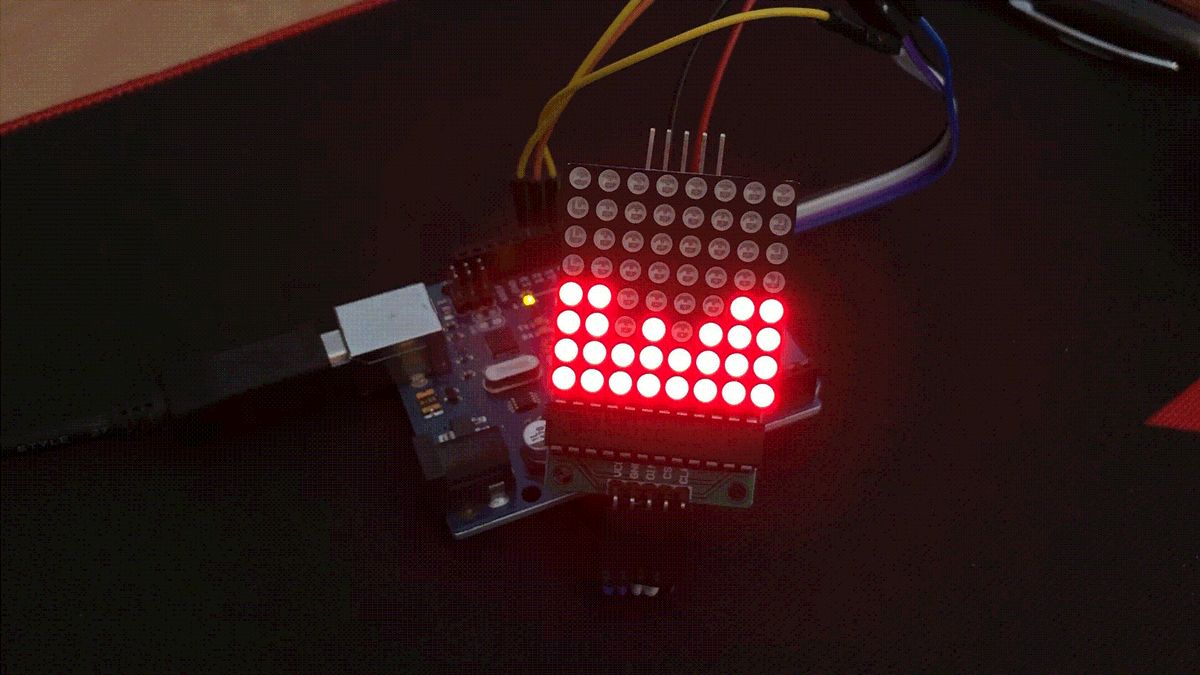# Open fire on an LED matrix displayIt is winter and the right time for warm thoughts. May this LED matrix open fire help.

## Circuit

The LED matrix is connected directly to the Arduino. This requires three digital pins for SPI communication and two cables for the power supply (GND and 5V +).

You can find more information on how the LED matrix works in the Tutorials section: Arduino Matrix Display 8 × 8 pixels and lots of fun.

## Code

Here is the program code. The light height of the individual rows is determined.

``````if (int(random(1)) == 0) {
for (int x = 0; x < 8; x++) {
theHeightTarget[x] = int(random(5)) + 1;
}
}``````

Then the program tries to adjust the current light height.

``````  // update
for (int x = 0; x < 8; x++) {
if (theHeightTarget[x] > theHeightCurrent[x])theHeightCurrent[x]++;
if (theHeightTarget[x] < theHeightCurrent[x])theHeightCurrent[x]--;
}``````

This is then shown on the matrix.

``````  // show display
for (int x = 0; x < 8; x++) {
for (int y = 0; y < theHeightCurrent[x]; y++) {
lc.setLed(0, x, y, 1);
}
for (int y = theHeightCurrent[x]; y < 8; y++) {
lc.setLed(0, x, y, 0);
}
}``````

Every now and then a spark is released and flies upwards.

``````// sparks
sparkX = int(random(8));
if (int(random(40)) == 0) {
for (int y = 4; y < 8; y++) {
if (y == 4) {
lc.setLed(0, sparkX, y, 1);
} else {
lc.setLed(0, sparkX, y, 1);
lc.setLed(0, sparkX, y - 1, 0);
}
delay(25);
}
} else {
delay(100);
}``````

Find the complete code her:

``````#include "LedControl.h"
LedControl lc = LedControl(12, 11, 10, 1);

int theHeightTarget;
int theHeightCurrent;

int fireHeight = 0;
int sparkX = 0;

void setup() {
lc.shutdown(0, false);
lc.setIntensity(0, 8);
lc.clearDisplay(0);
Serial.begin(115200);
}

void loop() {
//lc.clearDisplay(0);
// set Heights
if (int(random(1)) == 0) {
for (int x = 0; x < 8; x++) {
theHeightTarget[x] = int(random(5)) + 1;
}
}

// update
for (int x = 0; x < 8; x++) {
if (theHeightTarget[x] > theHeightCurrent[x])theHeightCurrent[x]++;
if (theHeightTarget[x] < theHeightCurrent[x])theHeightCurrent[x]--;
}

// show display
for (int x = 0; x < 8; x++) {
for (int y = 0; y < theHeightCurrent[x]; y++) {
lc.setLed(0, x, y, 1);
}
for (int y = theHeightCurrent[x]; y < 8; y++) {
lc.setLed(0, x, y, 0);
}
}

// spark – Funkenflug
sparkX = int(random(8));
if (int(random(40)) == 0) {
for (int y = 4; y < 8; y++) {
if (y == 4) {
lc.setLed(0, sparkX, y, 1);
} else {
lc.setLed(0, sparkX, y, 1);
lc.setLed(0, sparkX, y - 1, 0);
}
delay(25);
}
} else {
delay(100);
}
}``````